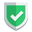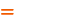## 下载修改`jsencrypt`

• `navigator.appName`
• `window.`

``````// if (j_lm && (navigator.appName == "Microsoft Internet Explorer")) {
//     BigInteger.prototype.am = am2;
//     dbits = 30;
// }
// else if (j_lm && (navigator.appName != "Netscape")) {
//     BigInteger.prototype.am = am1;
//     dbits = 26;
// }
// else { // Mozilla/Netscape seems to prefer am3
//     BigInteger.prototype.am = am3;
//     dbits = 28;
// }
BigInteger.prototype.am = am3;
dbits = 28;``````

``````// if (window.crypto && window.crypto.getRandomValues) {
//     // Extract entropy (2048 bits) from RNG if available
//     var z = new Uint32Array(256);
//     window.crypto.getRandomValues(z);
//     for (t = 0; t < z.length; ++t) {
//         rng_pool[rng_pptr++] = z[t] & 255;
//     }
// }
// sunan modify
var getRandomValues = function (array) {
for (var i = 0, l = array.length; i < l; i++) {
array[i] = Math.floor(Math.random() * 256);
}
return array;
}
var z = new Uint32Array(256);
getRandomValues(z);``````

``````//sunan modify
// if (window.removeEventListener) {
//     window.removeEventListener("mousemove", onMouseMoveListener_1, false);
// }
// else if (window.detachEvent) {
//     window.detachEvent("onmousemove", onMouseMoveListener_1);
// }
// return;``````

``````//sunan modify
// }
// else if (window.attachEvent) {
//     window.attachEvent("onmousemove", onMouseMoveListener_1);
// }``````

``````//sunan modify
//window.JSEncrypt = JSEncrypt;``````

## 引入`jsencrypt`

``````exports.JSEncrypt = JSEncrypt;
exports.default = JSEncrypt;``````

``const {JSEncrypt} = require('../../libs/jsencrypt');``

## 使用方式

newsn.net：这里是【评论】可见内容

• 注意替换上述代码里面的`<public_key>`字样。
• 上述代码中的`JSEncrypt`类名，来自于最开始的`import`

## 总结如果本文对您有帮助，或者节约了您的时间，欢迎打赏瓶饮料，建立下友谊关系。本博客不欢迎：各种镜像采集行为。请尊重原创文章内容，转载请保留作者链接。【加群】加入QQ群【175454274】和大家一起讨论这个问题【源码】本文代码片段及相关软件，请点此获取【绝密】秘籍文章入口，仅传授于有缘之人百度    smartapp